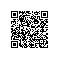# [LintCode] Candy 分糖果问题

There are N children standing in a line. Each child is assigned a rating value.

You are giving candies to these children subjected to the following requirements:

• Each child must have at least one candy.

• Children with a higher rating get more candies than their neighbors.

What is the minimum candies you must give?

Example

Given ratings = [1, 2], return 3.

Given ratings = [1, 1, 1], return 3.

Given ratings = [1, 2, 2], return 4. ([1,2,1]).

LeetCode上的原题，请参见我之前的博客Candy

class Solution {
public:
/**
* @param ratings Children's ratings
* @return the minimum candies you must give
*/
int candy(vector<int>& ratings) {
if (ratings.empty()) return 0;
int res = 0, n = ratings.size();
vector<int> dp(n, 1), dp2 = dp;
for (int i = 1; i < n; ++i) {
if (ratings[i] > ratings[i - 1]) dp[i] = dp[i - 1] + 1;
else dp[i] = 1;
}
for (int i = n - 2; i >= 0; --i) {
if (ratings[i] > ratings[i + 1]) dp2[i] = dp2[i + 1] + 1;
else dp2[i] = 1;
}
for (int i = 0; i < n; ++i) {
res += max(dp[i], dp2[i]);
}
return res;
}
};使用钉钉扫一扫加入圈子
+ 订阅